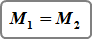1. Home
2. Computational Methods
3. Supercritical Flow Profiles

# Supercritical Flow Profiles

Culvert Studio has the ability to compute supercritical flow profiles with hydraulic jumps automatically. During friction loss calculations, if the energy equation cannot balance, the software reverses the calculation procedure, i.e. from upstream to downstream, and computes the supercritical profile.

It should be noted that Culvert Studio does not compute supercritical flow profiles for elliptical or arch shaped barrels. In these cases, critical depth is assumed.

## Hydraulic Jump

The Momentum Principle is used for determining depths and locations of hydraulic jumps. At each step (one tenth of the culvert length) during supercritical flow calculations, Culvert Studio computes the momentum and compares it to the momentum developed during the subcritical profile calculations. If the two momentum’s equal, it is established that a hydraulic jump must occur. There may be occasions when a hydraulic jump does not exist or is submerged.

The condition which must be satisfied if a hydraulic jump is to occur is when:Momentum, M1, of the subcritical profile equals the momentum, M2, of the supercritical profile.

Where:Where:

Q = flow rate
A = cross-sectional area of flow
Y = distance from the water surface to the centroid of A

The location of the jump is the point along the culvert barrel when M1 = M2 and is reported as the distance from the downstream end of the barrel.

The length of the jump however is difficult to determine, especially in circular channel sections. There have been many experimental investigations which have yielded results which are contradictory. Many have generalized that the jump length is somewhere between 4 and 6 times the Sequent depth. Culvert Studio assumes 5.